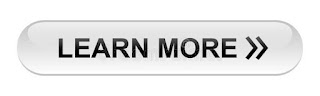222

## \$show=home

### Online Accounting Course  Achieve one of the highest distinctions in the accounting management profession: Become a Online Accountant. As an Online Accountant, you will have the knowledge and the skills to thrive in the competitive accounting industry. The Online Accounting course gives you expertise and real-world skills in accounting analysis. Join more than 100,000 professionals who have been recognized globally for their commitment to ethics and professionalism.## Latest \$type=blogging\$count=10\$author=hide\$comment=hide\$label=hide\$show=home\$date=hide

Measurement of cost of capital means to calculate the exact cost of capital. For example, there may be different rates of cost of capital between 2%  to 30%. But for knowing what is the exact rate of any source of finance, we measure cost of capital with simple formulas.

Measurement of cost of capital is totally related with the risk level of business. In above screenshot, you can see, when the risk is very very low. We will pay low cost of capital. Our measuring tool will show also low rate of this cost. Both cost of debt and cost of equity will low. If we have to simplify, we may calculate the weighted average cost of capital. It will also be very low if risk are at low level. But when the risk level of business will increase, we have to pay more cost of capital and rate angle will move to right side in measurement tool.

Because, we have explained all the formulae for calculating cost of capital, so this content, we will explain some examples of measuring cost of capital.

1. Cost of Debt Measurement

B Ltd issues \$ 1,00,000 9% debentures at a premium of 10%. The cost of flotation are 2%. The tax rate applicable is 60%. Measure cost of debt capital.

Kda = Interest /NP X (1-t)

NP = (1,00,000 + 10,000) - (1,10,000 X 2/100)  = 1,10,000 - 2,200 = 1,07,800

t = Tax rate

= 1,00,000 X 9% / 1,07,800 X ( 1- 0.6) = 3.34%

2. Cost of Preference Share Capital Measurement

A company issues 1000 7% preference shares of \$ 100 each at a premium of 10% redeemable after 5 years at par. Measure the cost of preference capital

Kpr = D + 1/n ( MV - NP ) / 1/2 ( MV + NP) X 100

D = 100,000 X 7 % = 7,000

MV = 1,00,000

NP = 1,00,000 + 1,00,000 X 10% = 1,10,000

n = 5 years

= 7,000 +1/5 ( 1,00,000 - 1,10,000

3. Cost of Equity Share Capital Measurement

A company is considering an expenditure of \$ 60 lakhs for expanding its operations. The relevant information is as follows.

Number of existing equity shares = 10 Lakhs
Market Value of existing share = \$ 60
Net Earnings = \$ 90 Lakhs

Measure the cost of existing equity share capital of new equity capital assuming that new shares will be issued at a price of \$ 52 per share and the cost of new issue will be \$ 2 per share.

Cost of existing equity share capital

Ke = EPS / MP

EPS = Earning per share

= \$ 90/ 10 = \$ 9

Ke = 9/60 X 100 = 15%

Cost of New Equity Share Capital

Ke = EPS / NP

= 9/ (52-2) X 100 = 18%

4. Cost of Retained Earning Measurement

A company's return available to shareholders is 15%. The average tax rate of shareholders is 40% and it is expected that 2% is brokerage cost that shareholders will have to pay while investing their dividends in alternative securities. What is the cost of retained earnings?

Cost of Retained Earnings = Kr = Ke ( 1- t) (1- b)

Ke = rate of return available to shareholders

t= tax rate
b = brokerage cost

Kr = 15% ( 1-0.4) (1- 0.02)

= 15% X 0.6 X 0.98

= 8.82%Name

ltr
item
Accounting Education: Measurement of Cost of Capital
Measurement of Cost of Capital
http://3.bp.blogspot.com/-SId8MJVQFTw/UcTRlaeaw3I/AAAAAAAALp0/3UKlWH6gccc/s640/cost+of+capital.PNG
http://3.bp.blogspot.com/-SId8MJVQFTw/UcTRlaeaw3I/AAAAAAAALp0/3UKlWH6gccc/s72-c/cost+of+capital.PNG
Accounting Education
https://www.svtuition.org/2013/06/measurement-of-cost-of-capital.html
https://www.svtuition.org/
https://www.svtuition.org/
https://www.svtuition.org/2013/06/measurement-of-cost-of-capital.html
true
2410664366776677676
UTF-8